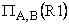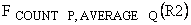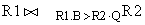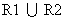Time: 3 Hours                                                                                                     Max. Marks: 100

NOTE: There are 9 Questions in all.

·      Question 1 is compulsory and carries 20 marks. Answer to Q. 1. must be written in the space provided for it in the answer book supplied and nowhere else.

·      Out of the remaining EIGHT Questions answer any FIVE Questions. Each question carries 16 marks.

·      Any required data not explicitly given, may be suitably assumed and stated.

Q.1       Choose the correct or best alternative in the following:                                         (2x10)

a.       The users who use easy-to-use menu are called

(A)  Sophisticated end users.               (B)  Naïve users.

(C)    Stand-alone users.                       (D) Casual end users.

b.      Which database level is closest to the users?

(A)    External                                        (B)  Internal

(C)  Physical                                        (D)  Conceptual

c.   Which are the two ways in which entities can participate in a relationship?

(A)    Passive and active                        (B)  Total and partial

(C) Simple and Complex                     (D)  All of the above

d.   The result of the UNION operation between R1 and R2 is a relation that includes

(A)    all the tuples of R1

(B)    all the tuples of R2

(C)  all the tuples of R1 and R2

(D)  all the tuples of R1 and R2 which have common columns

e.   Which of the following is a comparison operator in SQL?

(A)     =                                                 (B)  LIKE

(C)  BETWEEN                                  (D)  All of the above

f.    A set of possible data values is called

(A)     attribute.                                      (B)  degree.

(C)  tuple.                                            (D)  domain.

g.   Which of the operations constitute a basic set of operations for manipulating relational data?

(A)     Predicate calculus                         (B)  Relational calculus

(C)  Relational algebra                         (D)  None of the above

h.   Which of the following is another name for weak entity?

(A)    Child                                            (B) Owner

(C) Dominant                                      (D) All of the above

i.    Which of the following database object does not physically exist?

(A)   base table                                     (B) index

(C) view                                              (D) none of the above

j.    NULL is

(A)    the same as 0 for integer

(B)    the same as blank for character

(C)    the same as 0 for integer and blank for character

(D)    not a value

Answer any FIVE Questions out of EIGHT Questions.

Each question carries 16 marks.

Q.2     a.   What three main types of actions involve databases?  Briefly discuss these.          (6)

b.   What are the situations when DBMS should not be used?                                   (6)

c.   What is the difference between a database schema and a database state?            (4)

Q.3     a.   With the help of a diagram describe the typical component modules of a DBMS.                (8)

b.   Define the following:

(i)   Participation role                           (ii)   Recursive relationship type

(iii) Composite attribute                        (iv)  Entity                                               (8)

Q.4     a.   A database is to be constructed to keep track of the teams and games of a sport league.  A team has a number of players, not all of whom participate in each game.  It is desired to keep track of the players participating in each game of each team and the result of the game.

Create an ER diagram, completely with attributes, keys and constraints, for the above description.  State any assumptions that you make.                  (8)

b.   Discuss the types of integrity constraints that must be checked for the update operations – Insert and Delete.  Give examples.                                    (8)

(i)                  Primary and secondary storage.

(ii)                Open addressing and chaining for collision resolution.

(iii)               Spanned and unspanned records.

(iv)              Unordered and ordered file.                                                     (16)

Q.6     a.   What is recursive closure?  Why is it not possible to define this operation in relational algebra?                                                                   (4)

b.   Consider the two tables R1 (A,B,C) and R2 (P,Q,R) shown below

R1

 A B C a 15 10 a 25 8 b 15 5

R2

 P Q R b 15 5 c 10 8 b 5 6

Show the results of the following operations:

(i)(ii)(where F is the aggregate function)

(iii)(iV)(12)

Q.7     a.   Explain the EXISTS and UNIQUE functions of SQL.  Give an example for each.               (6)

b.   What is NULL?  Give an example to illustrate testing for NULL in SQL.             (4)

c.   Describe substring comparison in SQL.  For the relation Person(name, address), write a SQL query which retrieves the names of people whose name begins with ‘A’ and address contains ‘Bangalore’.         (6)

Q.8     a.   Consider the following relations with keys underlined

Street (name, location, city)

House (number, street_name)

Lives (name, house_number)

Define the above relations as tables in SQL making real world assumptions about the type of the fields.  Define the primary keys and the foreign keys.                                                     (7)

b.   For the above relations answer the following queries in SQL

(i)                  Get the names of persons who live in the street named ‘Mahatma Gandhi’.

(ii)                Get the house numbers street wise.

(iii)               Get the numbers of houses which are not occupied.                                (9)

Q.9     a.   How is the database organised in Oracle?                                                           (8)

b.   Explain the following with respect to QBE

(i)                  .CNT.

(ii)                I.

(iii)               P.

(iv)              D.                                                                                                          (8)